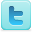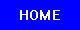Statistics & Probability
Science Fair Projects
Ideas and Sample Projects by Grade Level

Elementary School - Grades 4-6
P=Project   E=Experiment
A statistical estimation of the No. of headwords in a dictionary and the size of your vocabulary. [E]
Prove whether or not the birthday paradox holds true by looking at random groups of 23 or more people. [E]
Determine what happens when a test with two equally-likely outcomes is performed only a small number of times. [E]
Estimate the number of beans in small, medium, and large populations to test if estimates are more accurate for small or large populations. [E]
Test if the probability of drawing a particular card from a deck depends upon the number of that type of card in the deck. [E]
Determine if the shape of a die affects the fairness of the roll. [E]
The Effect of Number of Sides on the Fairness of a Die (Dice) [E]
Determine if flipping a coin is truly random. [E]
Determine if odds-makers' predictions about sporting events ere accurate. [E]
How many times do you have to drop ten quarters, while re-dropping all the ones that land on heads until you get all tails? [E]
An unbiased college football division 1A ranking system [E]
Do traffic signals decrease the amount of auto accidents? [E]
How accurately the egg producers measure their eggs? [P] [P]
Making estimations in measurement [E]
Have popular books changed to use simpler language over the past century? [E]
Middle / High School - Grades 7-12
P=Project   E=Experiment
Measure the distance balls travel when launched from a catapult, and use the results to construct a histogram and assess the catapult's reliability. [E]
Study the basic theory of combinatorial games using the game of Nim as an example [P]
Semantic Image Retrieval: Learning Gaussian Mixture Models of Semantic Concepts using Expectation-Maximization [E]
A simple statistical analysis of the frequency of colors of M&M's in a bag [E]
A Probability Problem with Theoretical Solution and Monte Carlo Simulations [E]
Study the variants of the Josephus Problem and properties of the graphs produced by these variants. [P]
Prove the best strategy for playing Hi-Lo using basic probability. [E]
A Combinatorial Interpretation of the Homotopy Groups of Finite Topologies [E]
Is Mathematics Discovered or Invented? [E]
Test the probabilities of rolling certain combinations of dice in roll-playing games. [E]
The Chances of Guessing Correctly on a Multiple Choice Exam [P] [P]
See if the lottery numbers are predictable. [E]
Determine if the Monty Hall Theory, created by Marilyn vos Savont, is mathematically correct. [E]
The Randomness of Card Shuffling: Manual vs. Automatic [E]
Can a Computer Generate Random Numbers Accurately? [E]
Determine if it is reasonable in Blackjack to act differently with a 2-card 16 than with a 3-card 16 against a dealers 10 [E]
Determine whether a particular area surveyed statistically represents the slightly larger area surrounding it. [E]
Determine if the probability of picking the right object is better by switching your initial choice with a variant of the shell game, where one choice that is for sure wrong is removed by the person in charge and shown to you after you make your first guess. [E]

Do Random Number Generators Follow Benford's Law? [E]
Determine whether multiplication, exponentiation, or addition will force a set of random numbers [0,1] to conform to Benford's Law. [E]
How large a survey sample must be to get an accurate representation of a whole population? [E] [E]
Pascal like Triangle, Sierpinski like Gaskets and Fibonacci like Sequences. [P]
What would happen with the use of different base numbers in a Debruijn Sequence? [E]
A pattern of numbers that involve radicals and Pascal's triangle. [E]
A Comparison of transect and radial sampling methods [E]
Comparing methods of biostatistical sampling [E]
Is there a correlation between money spent on middle school students and their performance in high school? [E]
Determine if the use of a Gaussian probability device actually follows a repeatable, predictable model of a bell curve. [E]
Streaks in baseball: A matter of chance? [E]
Which method of bioassay, radial sampling or transect-line sampling, will prove to be a more accurate representation of the whole population sampled. [E]
Can people choose truly random numbers? [E]
Discover if the normal curve applies to sets of large data. [E]
How do the dimensions of a baseball stadium affect batting statistics. [E]
Is there such a thing as streakiness in baseball? [E]
Predictive Analysis using Linear Regression [E]
The validity of Benford's Law and how it can be applied in real life situations. [E]
Test Benford's Law and Zipf's Law, to see if they actually work. [E]
R=Resource
Science Fair Projects Resources [R]
Citation Guides, Style Manuals, Reference [R]

 Mathematics Projects Math Topics Related Subjects Statistics & Probability Geometry & Trigonometry Applied Mathematics Games & Game Theory Number Theory Mathematics Learning Algebra & Calculus Computer Science Intelligence

 Science Fair Project Guide Home Science Fair Project Types The Scientific Method - How to Experiment The Display Board Topics, Ideas, Sample Projects